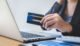The Capital Asset Pricing Model (CAPM) establishes a linear relationship between the required rate of return of a security and its systematic or un-diversifiable risk or beta. CAPM a model use to determine a theoretically appropriate required rate of return of an asset, to make decisions about adding assets to a well-diversified portfolio. As well as, CAPM enables us to be much more precise about how trade-offs between risk; and, return determine in the financial markets. So, what is the question; What does mean Capital Asset Pricing Model (CAPM)?

Contents:

## Here are explain What is the Capital Asset Pricing Model (CAPM)? with Meaning and Definition.

In CAPM the expected rate of return can also think of as a required rate of return because the market is assumed to be in equilibrium. Also, the Expected Rate of Return is the return that an investor expects to earn. Required Rate of Return of security the minimum expected the rate of return needed to induce an investor to purchase it.

1] According to A,

“CAPM is a method of determining the fair value of an investment based on the time value of money and the risk incurred.”

2] According to B,

“CAPM is a model used to determine a theoretically appropriate required rate of return of an asset, to make decisions about adding assets to a well-diversified portfolio.”

3] According to C,

“CAPM is used to estimate the fair value of high-risk stock and security portfolios by linking the expected rate of return with risk.”

Capital asset pricing model (CAPM) is a model that establishes a relationship between the required return and the systematic risk of an investment. As well as, It estimates the required return as the sum of the risk-free rate; and, the product of the security’s beta coefficient and equity risk premium. Also, Investors face two kinds of risks: systematic risk and unsystematic risk. As well as, Systematic risk is the risk of the whole economy or financial system [Hindi] going down and causing low or negative returns.

Cost of Capital: Meaning, Classification, and Importance!
##### For example;

The risk of recession, enactment of unfavorable regulation, etc. Systematic risk can’t avoid adding more investments to the portfolio (i.e. diversification) because a downturn in the whole economy affects all investments.

Unsystematic risk, on the other hand, is the risk specific to a particular investment. For example, unfavorable court ruling affecting the company, major disruption in the company’s supply chain, etc. Such risks can mitigate by adding additional investments to a portfolio. For example, a portfolio of 100-stocks is less prone to the negative performance of one company due to any specific event affecting it.

### CAPM calculate according to the following formula:

Rrf + [Ba x (Rm – Rrf) ] = Ra

Where in:

• Ra = Expected return on a security.
• Rrf = Risk-free rate.
• Ba = Beta of the security, and.
• Rm = Expected return on the market.

Since unsystematic risk can eliminate through diversification; Also, the capital asset pricing model doesn’t provide any reward for taking such a risk. It measures the required return based on the level of systematic risk inherent in a particular investment.